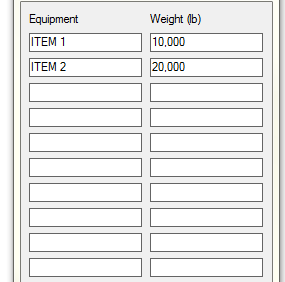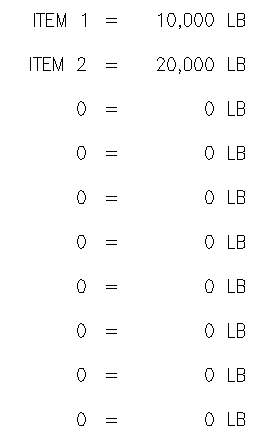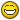# DCL Ignore Blank Values MText## Recommended PostsI am trying to write a routine that allows users to input items and weights in a dialog box, and outputs a formatted MText (among other things). I allow the user to input up to 10 items, but I only want the MText to use the values that are filled in.

Here's what my dialog box looks like:And here's what my output looks like:Here's my code for the Mtext:

```(setq val (strcat "\\pxtr18,c20,r30;\t" eq1 "\t=\t" wt1 " LB
\\P\t" eq2 "\t=\t" wt2 " LB
\\P\t" eq3 "\t=\t" wt3 " LB
\\P\t" eq4 "\t=\t" wt4 " LB
\\P\t" eq5 "\t=\t" wt5 " LB
\\P\t" eq6 "\t=\t" wt6 " LB
\\P\t" eq7 "\t=\t" wt7 " LB
\\P\t" eq8 "\t=\t" wt8 " LB
\\P\t" eq9 "\t=\t" wt9 " LB
\\P\t" eq10 "\t=\t" wt10 " LB"

))

(entmake
(list
(cons 0 "MTEXT")
(cons 100 "AcDbEntity")
(cons 100 "AcDbMText")
(cons 10 pt1)
(cons 1 val)
(cons 8 "NOTES")
(cons 40 0.09375)
(cons 7 "ROMANS")
(cons 41 4)))```

Any suggestions how to make it ignore the "0" values?

##### Share on other sitesIn the string concatenation, you are simply taking values regardless. Use conditionals (on your "eq"s) so that you only include lines that actually have entries.

##### Share on other sitesThanks neophoible, here's how I solved it.

```(if (/= eq1 "0") (setq a (strcat "\\P\t" eq1 "\t=\t" wt1 " LB")) (setq a ""))
(if (/= eq2 "0") (setq b (strcat "\\P\t" eq2 "\t=\t" wt2 " LB")) (setq b ""))
(if (/= eq3 "0") (setq c (strcat "\\P\t" eq3 "\t=\t" wt3 " LB")) (setq c ""))
(if (/= eq4 "0") (setq d (strcat "\\P\t" eq4 "\t=\t" wt4 " LB")) (setq d ""))
(if (/= eq5 "0") (setq e (strcat "\\P\t" eq5 "\t=\t" wt5 " LB")) (setq e ""))
(if (/= eq6 "0") (setq f (strcat "\\P\t" eq6 "\t=\t" wt6 " LB")) (setq f ""))
(if (/= eq7 "0") (setq g (strcat "\\P\t" eq7 "\t=\t" wt7 " LB")) (setq g ""))
(if (/= eq8 "0") (setq h (strcat "\\P\t" eq8 "\t=\t" wt8 " LB")) (setq h ""))
(if (/= eq9 "0") (setq i (strcat "\\P\t" eq9 "\t=\t" wt9 " LB")) (setq i ""))
(if (/= eq10 "0") (setq j (strcat "\\P\t" eq10 "\t=\t" wt10 " LB")) (setq j ""))

(setq val (strcat "\\pxtr18,c20,r30;\t" a b c d e f g h i j))```

##### Share on other sitesYou're welcome. Very good, very straightforward, gets the job done.I should have asked if you actually ran it to make sure it worked. The reason I'm mentioning it is that you may have a lot of expressions to evaluate after your initial "if". If I'm right, then an easy way to fix that would be to add a "progn" before them, that is, group them inside.

I think I just got confused by the indentation. I'm used to keeping expressions in line with others at the same level.

Edited by neophoible

## Join the conversation

You can post now and register later. If you have an account, sign in now to post with your account.
Note: Your post will require moderator approval before it will be visible.×   Pasted as rich text.   Restore formatting

Only 75 emoji are allowed.